﻿ A Two-Parameter Poisson-Sujatha DistributionAmerican Journal of Mathematics and Statistics

p-ISSN: 2162-948X    e-ISSN: 2162-8475

2020;  10(3): 70-78

doi:10.5923/j.ajms.20201003.02### A Two-Parameter Poisson-Sujatha Distribution

Rama Shanker 1, Kamlesh Kumar Shukla 2, Tekie Asehun Leonida 3

1Department of Statistics, Assam University, Silchar, India

2Department of Statistics, Mainefhi College of Science, Asmara, Eritrea

3Department of Applied Mathematics, University of Twente, The Netherlands

Correspondence to: Rama Shanker , Department of Statistics, Assam University, Silchar, India.
 Email: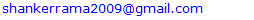Abstract

A two-parameter Poisson-Sujatha distribution which is a Poisson mixture of two-parameter Sujatha distribution, and includes Poisson-Sujatha distribution as particular case has been proposed. Its moments and moments based measures including coefficient of variation, skewness, kurtosis and index of dispersion have been obtained. Maximum likelihood estimation has been explained for estimating its parameters. Goodness of fit of the proposed distribution has been explained with two over-dispersed count datasets and the fit has been compared with one parameter Poisson-Lindley distribution and Poisson-Sujatha distribution and a generalization of Poisson-Sujatha distribution.

Keywords: Sujatha distribution, Poisson-Sujatha distribution, Two-parameter Sujatha distribution, Moments based measures, Maximum likelihood estimation

Cite this paper: Rama Shanker , Kamlesh Kumar Shukla , Tekie Asehun Leonida , A Two-Parameter Poisson-Sujatha Distribution, American Journal of Mathematics and Statistics, Vol. 10 No. 3, 2020, pp. 70-78. doi: 10.5923/j.ajms.20201003.02.

### 1. Introduction

In statistics literature, Poisson distribution is the common distribution for modeling count data. However, the unique feature of equality of the mean and the variance (equi-dispersed) of Poisson distribution makes it unsuitable for modeling count data which are under-dispersed (mean greater than variance) or over-dispersed (mean less than variance). In recent years, several researchers have proposed Poisson mixture of lifetime distributions which are useful for over-dispersed or under-dispersed. The over-dispersed Poisson mixed distributions are Poisson-Lindley distribution (PLD), a Poisson mixture of Lindley distribution of Lindley (1958) proposed by Sankaran (1970), Poisson-Sujatha distribution (PSD), a Poisson mixture of Sujatha distribution of Shanker (2016 a) introduced by Shanker (2016 b), some among others.
The probability density function (pdf) of Sujatha distribution having scale parameter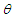and introduced by Shanker (2016 a) is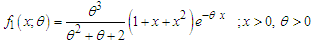(1.1)
Various properties including shapes of the density, moments and moments based measures, skewness, kurtosis, index of dispersion, hazard rate function, mean residual life function, stochastic ordering, mean deviation, stress-strength reliability, along with the estimation of parameter and applications for modeling lifetime data from biomedical science and engineering of Sujatha distribution are available in Shanker (2016 a). Kaliraja and Perarasan (2019) studied a stochastic model on the generalization of Sujatha distribution for the effects of two types of exercise on plasma growth hormone.
Shanker (2016 b) obtained Poisson-Sujatha distribution (PSD) by compounding Poisson distribution with Sujatha distribution. The PSD is defined by its probability mass function (pmf)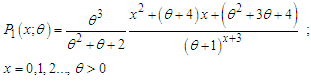(1.2)
Statistical properties including shapes of pmf, moments and moments based measures, over-dispersion, unimodality and increasing hazard rate, estimation of parameter and applications to model over-dispersed data have been discussed by Shanker (2016 b). Wesley et al (2018) proposed a zero-modified Poisson-Sujatha distribution to model over-dispersed count data and discussed its several important properties and applications.
Shanker et al (2017) proposed a generalization Sujatha distribution (AGSD) using convex combination of exponential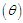, gamma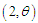and gamma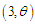distributions and defined by its pdf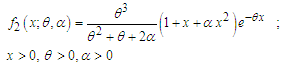(1.3)
where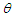is a scale parameter and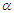is a shape parameter. Lindley distribution and Sujatha distribution are the particular cases of AGSD for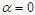and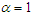, respectively. Various statistical properties, estimation of parameters and applications of AGSD have been discussed by Shanker et al (2017).
Shanker and Shukla (2019) derived a generalization of Poisson-Sujatha distribution (AGPSD) by mixing Poisson distribution with AGSD. The AGPSD is defined by its pmf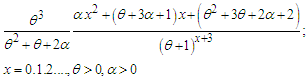(1.4)
It can be easily shown that Poisson-Lindley distribution (PLD of Sankaran (1970) and PSD of Shanker (2016 b) are special cases of AGPSD for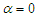and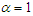, respectively. Statistical properties based on moments, unimodality and increasing hazard rate, estimation of parameter and applications of AGPSD have been studied by Shanker and Shukla (2019).
Mussie and Shanker (2018) proposed a two-parameter Sujatha distribution (TPSD) defined by its pdf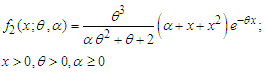(1.3)
where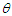is a scale parameter and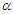is a shape parameter. It can be easily verified that (1.3) reduces to Sujatha distribution (1.1) and size-biased Lindley distribution (SBLD) for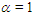and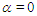respectively.
The main motivation for proposing a two-parameter Poisson-Sujatha distribution (TPPSD) are (i) Sujatha distribution is a better model than both exponential and Lindley distribution for modeling lifetime data, and PSD being a Poisson mixture of Sujatha distribution gives better fit than both Poisson and Poisson-Lindley distribution (PLD), and (ii) TPSD gives much better fit than exponential, Lindley and Sujatha distribution, it is expected that TPPSD being a Poisson mixture of TPSD would provide better fit over PLD, PSD and other discrete distributions.
Keeping these points in mind, a two-parameter Poisson-Sujatha distribution (TPPSD), a Poisson mixture of TPSD has been proposed and its moments and moments based measures have been obtained and their behaviors have been studied. Maximum likelihood estimation of TPPSD has been discussed for the estimation its parameters and its applications have been discussed with two examples of observed count datasets from ecology and demography.

### 2. A Two-Parameter Poisson-Sujatha Distribution

A random variable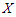is said to follow a two-parameter Poisson-Sujatha distribution (TPPSD) if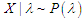and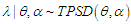. That is,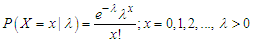, and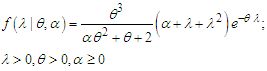The pmf of unconditional random variable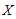can be obtained as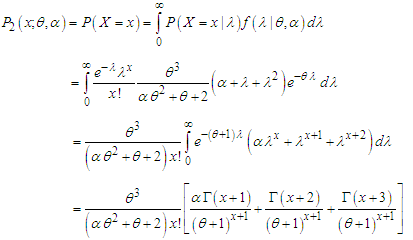(2.1)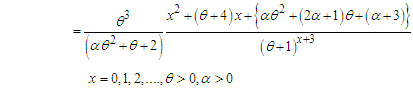(2.2)
We would call this two-parameter Poisson-Sujatha distribution (TPPSD) because for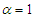, it reduces to one parameter PSD given in (1.2).
It can be easily shown that TPPSD is unimodal and has increasing hazard rate. Since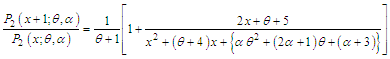is decreasing function in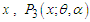is log-concave. Now using the results of relationship between log-concavity, unimodality and increasing hazard rate (IHR) of discrete distributions available in Grandell (1997), it can concluded that TPPSD has an increasing hazard rate and unimodal.
The behavior of the pmf of TPPSD for varying values of parameters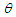and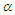are shown in figure 1.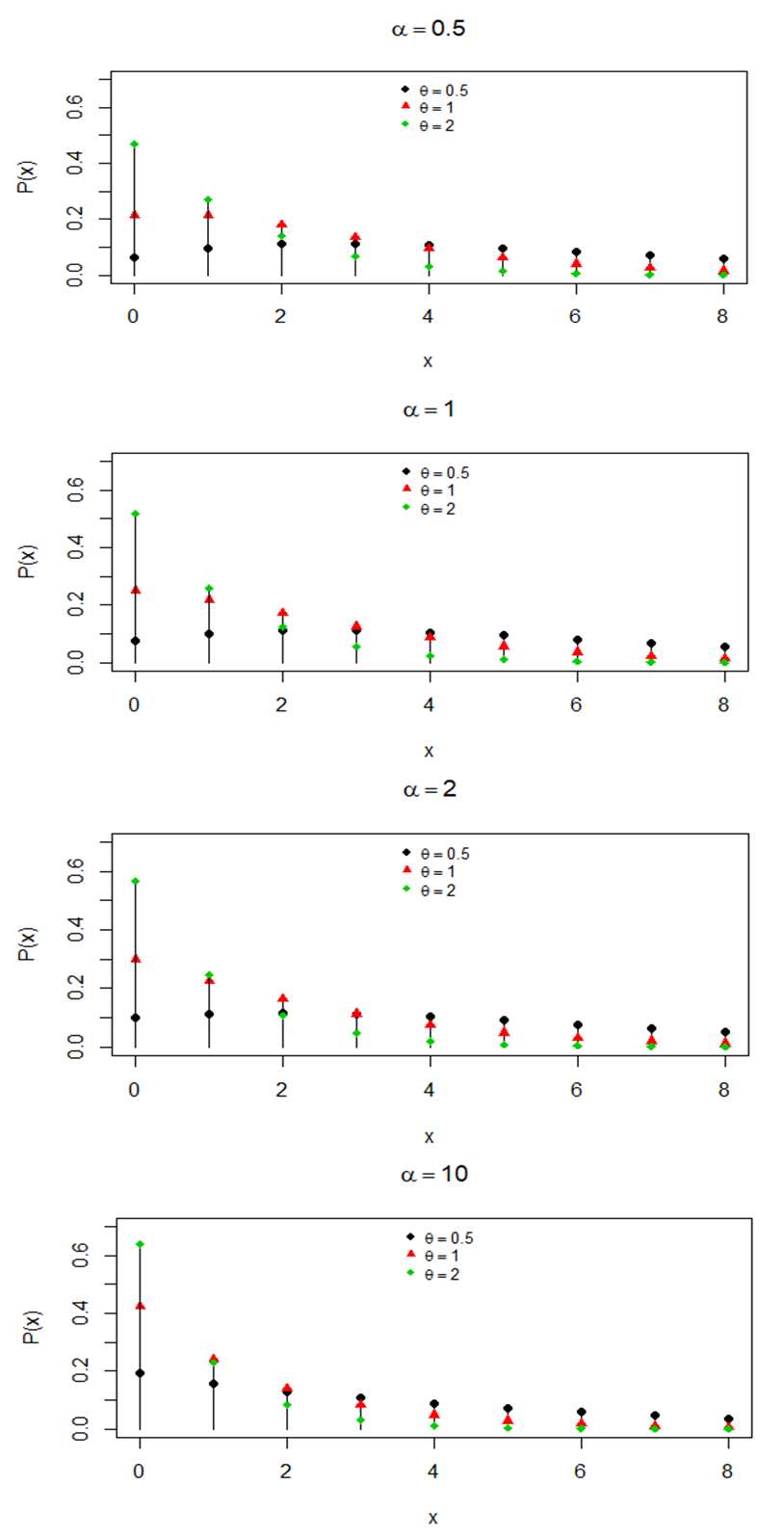Figure 1. Behaviour of pmf of TPPSD for varying values of parameters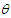and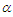### 3. Moments Based Measures

The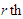factorial moment about origin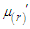of TPPSD (2.2) can be obtained as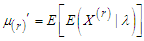, where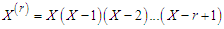. Using (2.1), the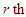factorial moment about origin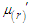of TPPSD (2.2) can be obtained as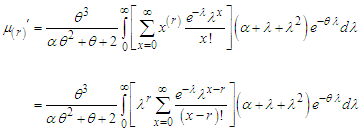Taking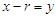within the bracket, we get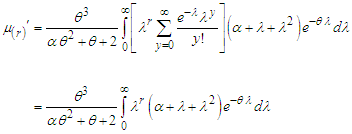After some tedious algebraic simplification, a general expression for the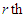factorial moment about origin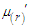of TPPSD (2.2) can be expressed as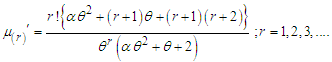(3.1)
It can be easily verified that at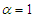, the expression (3.1) reduces to the corresponding expression of PSD. Substituting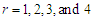in (3.1), the first four factorial moments about origin of TPSD can be obtained as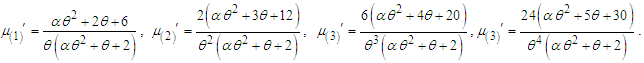Now using the relationship between factorial moments about origin and moments about origin, the first four moment about origin of the TPPSD are obtained as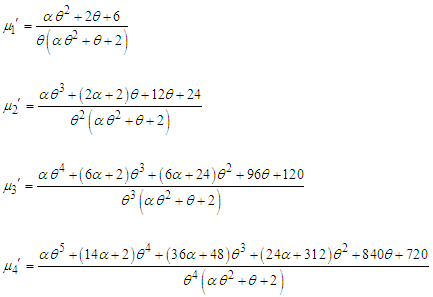Using the relationship between moments about mean and the moments about origin, the moments about mean of TPPSD are obtained as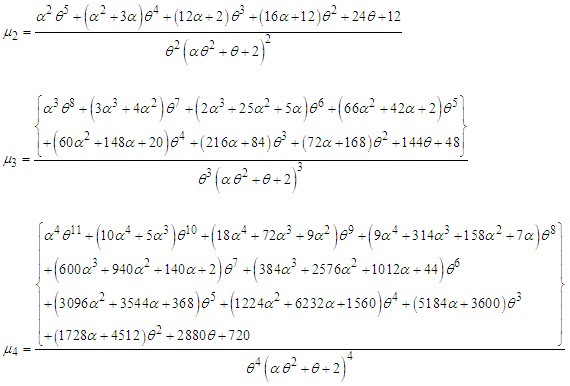The coefficient of variation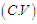, coefficient of Skewness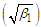, coefficient of Kurtosis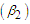, and index of dispersion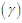of TPPSD are thus given by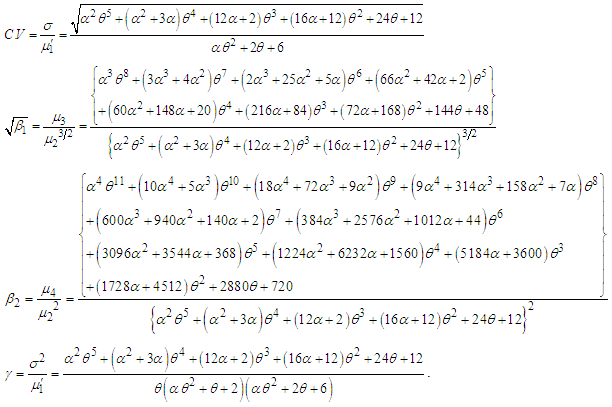It can be easily verified that at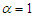, expressions of these statistical constants of TPPSD reduce to the corresponding expressions for PSD.
The behaviors of coefficient of variation (C.V), coefficient of skewness (C.S), coefficient of kurtosis (C.K) and index of dispersion (I.D) of TPPSD for varying values of parametersand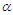have been explained through graphs and presented in figure 2.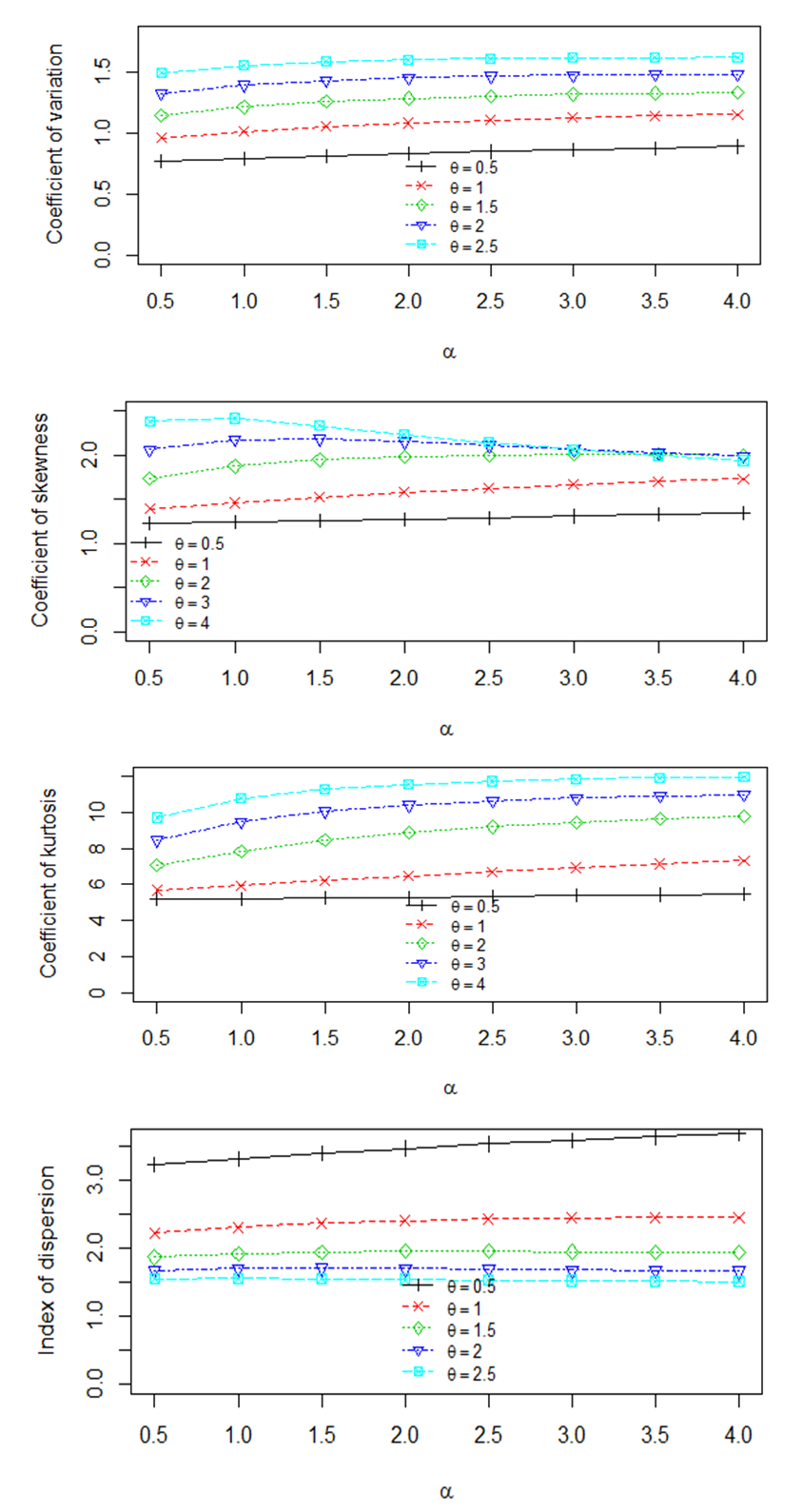Figure 2. Behaviors of coefficient of variation (C.V), coefficient of skewness (C.S), coefficient of kurtosis (C.K) and index of dispersion (I.D) of TPPSD for varying values of parameters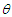and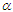### 4. Maximum Likelihood Estimation of Parameters

Suppose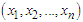be a random sample of size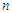from TPPSD and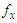be the observed frequency in the sample corresponding to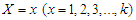such that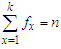, where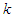is the largest observed value having non-zero frequency. The likelihood function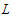of TPPSD is given by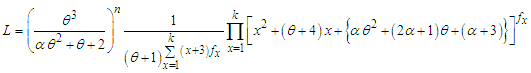The log likelihood function is thus obtained as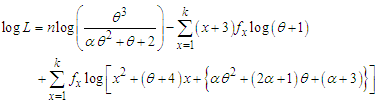The maximum likelihood estimates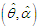of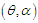of TPPSD is the solutions of the following log likelihood equations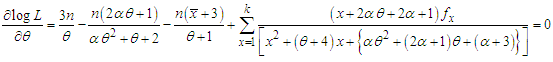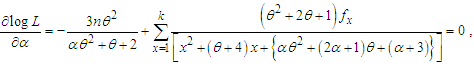where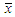is the sample mean. These two log likelihood equations do not seem to be solved directly because they do not have closed forms. Therefore, to find the maximum likelihood estimates of parameters an iterative method such as Fisher Scoring method, Bisection method, Regula Falsi method or Newton-Raphson method can be used. In this paper Newton-Raphson method has been used using R-software.
 Table 1. Observed and Expected number of European red mites on Apple leaves, available in Bliss (1953)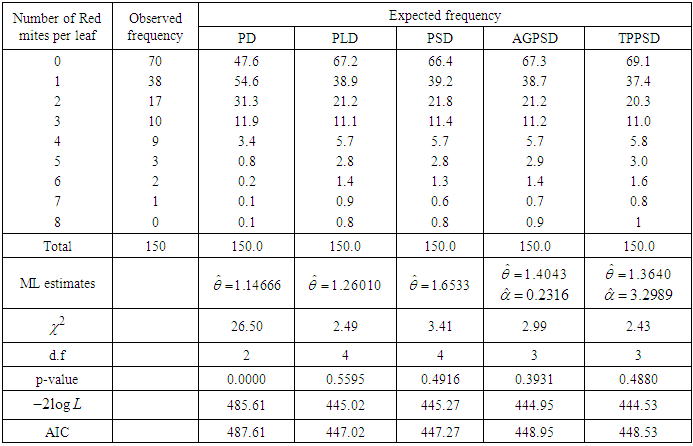Table 2. Observed and Expected number of households according to the number of male migrants aged 15 years and above, available in Shukla and Yadav (2006)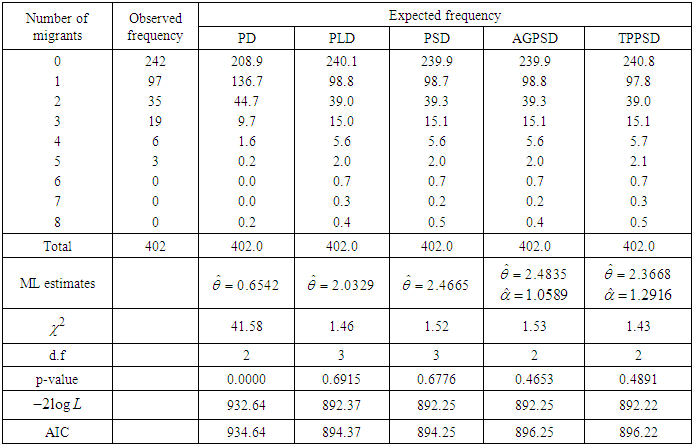### 5. Applications

The applications and the goodness of fit of the TPPSD have been demonstrated with two real count datasets. The first dataset is from ecology regarding the observed number of European red mites on Apple leaves, available in Bliss (1953) and the second dataset is from demography regarding the observed number of households according to the number of male migrants aged 15 years and above, available in Shukla and Yadav (2006). These two datasets are over-dispersed data. Maximum likelihood estimation has been used to fit Poisson distribution (PD), Poisson-Lindley distribution (PLD), PSD, AGPSD and TPPSD. The AIC (Akaike information criterion) has been calculated using the formula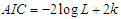, where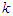is the number of parameters involved in the distribution. The distribution having less value of chi-square and AIC is the better distribution. Based on the values of chi-square and AIC of the considered distribution, it is obvious that TPPSD is competing well with the considered one parameter and two-parameter discrete distributions and, therefore, TPPSD can be considered an important two-parameter discrete distribution for ecology and migration data.

### 6. Concluding Remarks

In this paper, a two-parameter Poisson Sujatha distribution (TPPSD) by compounding Poisson distribution with two-parameter Sujatha distribution, which includes Poisson-Sujatha distribution (PSD) as a special case, has been proposed. The unimodality and increasing hazard rate properties of the distribution has been explained. Its moments and moments based measures have been derived. The nature of coefficients of variation, skewness, kurtosis and index of dispersion has been discussed with varying values of parameters. The method of maximum likelihood estimation has been discussed. The applications of the proposed distribution has been explained through two examples of count data, one from ecology and one from demography and its goodness of fit has been found quite satisfactory over PD, PLD, PSD, and AGPSD.

### ACKNOWLEDGEMENTS

Authors are grateful to the editor in chief of the journal and the anonymous reviewer for constructive comments which were helpful in improving the quality and the presentation of the paper.

### References

  Bliss, C.I. (1953): Fitting negative binomial distribution to biological data, Biometrics, 9, 177 – 200.  Grandell, J. (1997): Mixed Poisson Processes, Chapman& Hall, London.  Kaliraja, M. and Perarasan, K. (2019): A new stochastic model on the generalization of Sujatha distribution for the effects of two types of exercise on plasma growth hormone, The International Journal of Analytical and Experimental Model Analysis, 11(9), 1164 – 1170.  Lindley, D.V. (1958): Fiducial distributions and Bayes’ theorem, Journal of the Royal Statistical Society, Series B, 20, 102- 107.  Sankaran, M. (1970): The discrete Poisson-Lindley distribution, Biometrics, 26: 145-149.  Shanker, R. (2016 a): Sujatha distribution and Its Applications, Statistics in Transition new Series, 17 (3), 1 – 20.  Shanker, R. (2016 b): The discrete Poisson-Sujatha distribution, International Journal of Probability and Statistics, 5(1), 1- 9.  Shanker, R., Shukla, K.K., and Hagos, F. (2017): A Generalization of Sujatha distribution and its Applications to Real lifetime data, Journal of Institute of Science and Technology (JIST), 22(1), 77 – 94.  Shanker, R. and Shukla, K.K. (2019): A generalization of Poisson-Sujatha Distribution and Its Applications to Ecology, International Journal of Biomathematics, 12 (2), 1- 11.  Mussie, T. and Shanker, R. (2018): A Two-Parameter Sujatha distribution, Biometrics & Biostatistics International Journal, 7(3), 188 – 197.  Shukla, K.K. and Yadav, K.N.S. (2006): The distribution of number of migrants at household level, Journal of Population and Social Studies, 14(2), 153 – 166.  Wesley, B., Agelica, M.T.R., Katiane, S.C., Marinaho, G.A., and Francisco, L.N. (2018): On Zero-modified Poisson-Sujatha distribution to model over-dispersed data, Austrian Journal of Statistics, 47(3), 1 – 19.